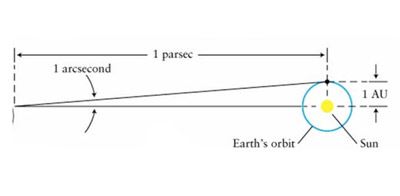A# arcsecond and arcminuteOne arcsecond is the angle subtended by the Earth's mean orbital radius at a distance of 1 parsec (= 3.26 light-years).

An arcsecond (arcsec or ") is an angular measurement equal to 1/3600 of a degree. There are 206,264.5" in a radian, so that 1" = 4.848 ×10–6 radians.

Turbulence in Earth’s atmosphere causes point sources, such as stars, to be smeared out and twinkle. The extent of this smearing out is referred as "seeing". Under the best atmospheric conditions, point sources will be spread out to an angular diameter of about 0.5”.

The trigonometric parallax of an object at a distance of 1 parsec (pc) is 1" (see diagram), however, there are no stars beyond the Solar System with parallaxes equal to or greater than 1". The nearest star system to the Sun, that of Alpha Centauri, lies about 1.3 pc away and has a parallax of 0.75".

A milliarcsecond = 10–3 arcsec. A microarcsecond (μas) = 10–6 arcsec.

An arcminute (arcmin or '), is an angular measurement equal to 1/60 of a degree or 60". There are 3,437.75' in a radian, so that 1' = 2.909 × 10–4 radians.

As seen from Earth, the Sun and Moon each have an angular diameter of about 30 arcmin.